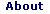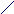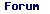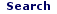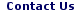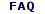Mon - 01 Jun 2020

WELCOME TO RFCABLES.ORG

## mW to dBm Calculator

### dBm Definition

dBm is defined as power ratio in decibel (dB) referenced to one milliwatt (mW). It is an abbreviation for dB with respect to 1 mW and the "m" in dBm stands for milliwatt.

dBm is different from dB. dBm represents absolute power, whereas dB is a ratio of two values and is used to represent gain or attenuation. For example, 3 dBm means 2 mW, and 3 dB means a gain of 2.Similarly, -3 dBm means 0.5 mW, whereas -3 dB means attenuation of 2.

The formula to calculate dBm from mW is: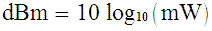mW Definition

Enter value to convert from mW to dBm below.

 Enter mW =dBm =(Value Rounded to 4 digits after decimal)

dBm to mW Calculator

milli-Watt to dBm Table

dBm to milli-Watt Table